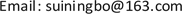1 μm波段零色散高非线性光子晶体光纤的设计<sup> </sup>1. 引言

2. 理论分析与光纤结构

∇ × [ 1 μ r ∇ × E ] = ( ω c ) 2 ε r × E (1)

D = − λ c d 2 Re ( n e f f ) d λ 2 (2)

γ = 2 π n 2 λ A e f f (3)

A e f f = ( ∬ E 2 d x d y ) 2 ∬ E 4 d x d y (4)

n 2 − 1 = 0.6961663 λ 2 λ 2 − 0.0684043 2 + 0.4079426 λ 2 λ 2 − 0.1162414 2 + 0.8974794 λ 2 λ 2 − 9.896161 2 (5)

3. 数值模拟和结果分析

3.1. 色散特性

3.2. 有效模场面积及非线性特性

4. 结论